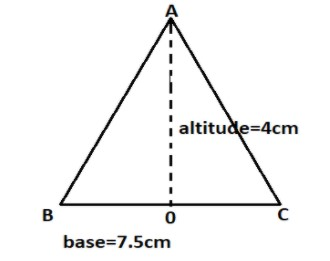Find the area, in square meters, of the triangle, whose base and altitude are:Base=7.5cm , altitude = 4cm.Verified
146.4k+ views
Hint: We will use the formula of area of the triangle to calculate the required value of the area of the triangle

Formula used: $\Delta = \dfrac{1}{2} \times base \times height$ to find the area of the triangle in square meters. Height and altitude are the same in a triangle.

Complete step by step solution:
We have to find the area of a triangle whose base is given as 7.5cm and altitude is given as 4cm. So, let the triangle be $\Delta ABC$ such that :Now we know that
Area of a $\Delta = \dfrac{1}{2} \times base \times height.$
Then, Area of $\Delta ABC = \dfrac{1}{2} \times base \times altitude$
Now in $\Delta ABC$ , base$BC = 7.5cm$ and altitude or height $OA = 4cm$ .
Next, we will put the values of the base and height of $\Delta ABC$ in the formula for Area of $\Delta$ and get:
Area of $\Delta ABC = \dfrac{1}{2} \times base \times altitude$
$Area Of\Delta ABC = \dfrac{1}{2} \times BC \times OA \\ Area Of\Delta ABC = \dfrac{1}{2} \times 7.5 \times 4 \\ Area Of\Delta ABC = 15c{m^2} \\$
So we will get an area of $\Delta ABC$=$= 15c{m^2}$ .
However the solution does not end here, since we need to find the area in square meters.
So we need to convert the area we have calculated from square centimeters to square meters.
Now,
$1c{m^2} = 1cm \times 1cm$
And,
$1cm = 0.01m \\ \because 100cm = 1m \\$
Then, if $1cm = 0.01m$,
$\Rightarrow 1c{m^2} = 1cm \times 1cm \\ = 0.01m \times 0.01m \\ = \dfrac{1}{{100}}m \times \dfrac{1}{{100}}m \\ = \dfrac{1}{{10000}}{m^2} \\ = 0.0001{m^2} \\$
If $1c{m^2} = 0.0001{m^2}$ then $15c{m^2}$ will be
$= 15 \times 0.0001{m^2} \\ = 0.0015{m^2} \\$
Therefore, the area of $\Delta ABC$ is $0.0015{m^2}$ , when expressed in square meters.
Hence area of the triangle with base =7.5cm and altitude = 4cm. is $0.0015{m^2}$.

Note:If the height of the triangle is not given and only the sides of the triangle are given, then we cannot use this formula. We will use Heron’s Formula to find the area of a triangle whose three sides are given to us.
For Heron’s formula first we will calculate ‘s’ which is the semi perimeter as :
$s = \dfrac{{a + b + c}}{2}$ , where a,b and c are the three sides of a triangle respectively. And then we will find the Area of $\Delta = \sqrt {s(s - a)(s - b)(s - a)}$ . This is Heron’s formula for finding the area of a triangle whose all sides are given to us.